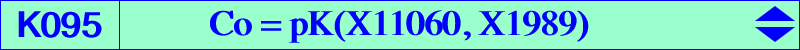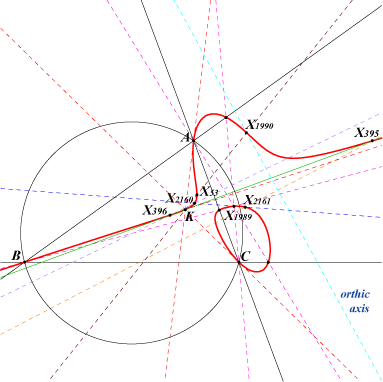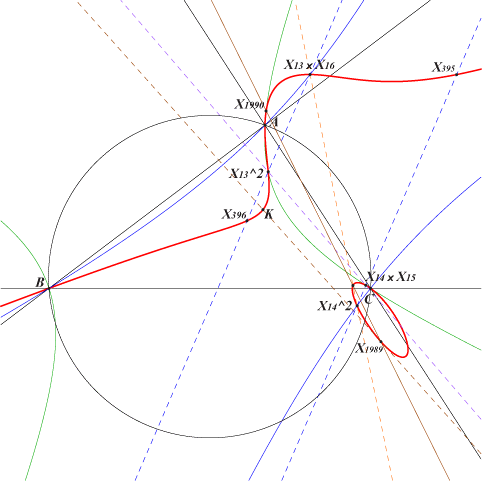X(6), X(53), X(395), X(396), X(1989), X(1990), X(2160), X(2161), X(11063), X(11069), X(11070), X(11071), X(11072), X(11073), X(11074), X(11075), X(11076), X(11077), X(11079), X(11080), X(11081), X(11082), X(11083), X(11084), X(11085), X(11086), X(11087), X(11088), X(11089) P1 = X(11077) = X(3) x X(1141) on the line X(53)X(1989) P2 = X(11063), tangential of K, X(1989)-Ceva conjugate of K P3 = X(11079), the isoconjugate of X(1990) X(11080) = X(13)^2, X(11085) = X(14)^2 : barycentric squares of the Fermat points X(11081) = X(13) x X(16), X(11086) = X(14) x X(15) : barycentric products. See other points, table and figure below. Ωa, Ωb, Ωc see Table 41 and CL052We meet the cubic Co in Special isocubics §6.1 and in the Orthopivotal cubics paper §6.2.1. Co is a pK with pivot Po = X(1989), isogonal conjugate of X(323) or the barycentric product of the Fermat points X(13) and X(14). Its pole is the barycentric product X(11060) of X(1989) and X(6), of X(2160) and X(2161) and more generally of each pair of points collinear with X(1989). See the collinearities below. Co is the isogonal transform of K856 = pK(X323, X2). These two cubics are anharmonically equivalent to the Neuberg cubic. See Table 20. K095 is the transform of the Neuberg cubic under the mapping f : u : v : w –> (SB v – SC w) / (c^2v^2 – b^2w^2) : : . See below and also the related Q053 and K678. The barycentric products of K261a by X(14) and K261b by X(13) are both K095. Hence, the barycentric quotients of K261a by X(13) and K261b by X(14) are both K856. Locus properties Locus of poles of all pK60+ i.e. pivotal stelloids. The locus of the pivots of these pK60+ is the Neuberg cubic and the locus of the isopivots is K060. The asymptotes concur at a point X on Q004. Locus of poles of all pivotal orthopivotal cubics or, equivalently, the locus of poles of all pivotal circular cubics passing through the Fermat points.The locus of the pivots is Kn = K060 and the locus of the isopivots is K001. The singular focus lies on Q041. The orthic lines of all these cubics pass through G. See K381 and K599 for analogous properties.The points mentioned above also lie on several remarkable conics.The points... lie on the circum-conic through... remarks X(13)^2, X(14)^2 X(4), X(30), X(477), X(523), X(1138), X(1990) rectangular hyperbola with perspector X(1637) X(13) x X(16), X(14) x X(15) X(30), X(74), X(186), X(526), X(1464), X(1511) isogonal transform of the asymptote of the Neuberg cubic, perspector X(30) x X(526) X(13)^2, X(14) x X(15) X(6), X(14), X(15), X(298), X(2378) barycentric product of the isogonal transform of the Fermat axis by X(14), perspector X(6137) X(14)^2, X(13) x X(16) X(6), X(13), X(16), X(299), X(2379) barycentric product of the isogonal transform of the Fermat axis by X(13), perspector X(6138) The points... lie on the in-conic through... remarks X(13)^2, X(14)^2 X(32) perspector X(23588) = X(476)^2Collinearities on K095 Recall that X(m) x X(n) is a barycentric product and X(n)2 is a barycentric square.X(1989), X(13)2 , X(14) x X(15) X(1989), X(14)2, X(13) x X(16) X(1989), X(13) x X(18), X(14) x X(62) X(1989), X(13) x X(61), X(14) x X(17) X(1990), X(13)2 , X(13) x X(61) X(1990), X(14)2 , X(14) x X(62) P1, P2, P3 P1, X(13) x X(61), X(14) x X(62) P2, X(13)2 , X(13) x X(18) P2, X(14)2 , X(14) x X(17) P2, X(13) x X(2380), X(14) x X(2381) P3, X(13) x X(16), X(14) x X(15) X(13) x X(16), X(14) x X(62), X(14) x X(2381) X(13) x X(61), X(13) x X(2380), X(14) x X(15) X(6), X(53), X(1990) X(6), X(395), X(396) X(6), X(13) x X(16), X(13) x X(61) X(6), X(14) x X(15), X(14) x X(62) X(53), X(1989), P1 X(53), X(13) x X(16), X(13) x X(18) X(53), X(14) x X(15), X(14) x X(17) X(395), X(1989), X(14) x X(2381) X(395), X(13) x X(18), X(13) x X(61) X(395), X(14)2 , X(14) x X(15) X(396), X(1989), X(13) x X(2380) X(396), X(13)2 , X(13) x X(16) X(396), X(14) x X(17), X(14) x X(62) X(1989), X(1990), P3 X(1989), X(2160), X(2161)The mapping f : M = u : v : w → f(M) = (SB v – SC w) / (c^2 v^2 – b^2 w^2) : : For M different of A, B, C, H and the in/excenters, consider the diagonal rectangular hyperbola (H) through M and the in/excenters. Let P be the pole of the line HM with regard to (H). The point f(M) is the barycentric product of H and P. Naturally, if N is the second intersection of the line HM and (H) then f(M) = f(N) = T. It follows that f is a (2,1) correspondence. *** Now, if T is given, the points M, N can be constructed as follows. Let S be the barycentric quotient T ÷ H and let S* be its isogonal conjugate. The line HS* contains M, N. The trilinear pole of the line KT is the center of the diagonal rectangular hyperbola (H) which meets the line HS* at the requested points M, N. These points are not necessarily real nor distinct. They coincide if and only if T lies on K678.   For more details and other properties see A Remarkable Rational Transformation related to Pivotal Cubics.